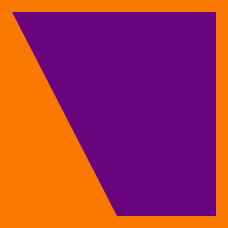Geometry

# Quadrilaterals: Level 4 Challenges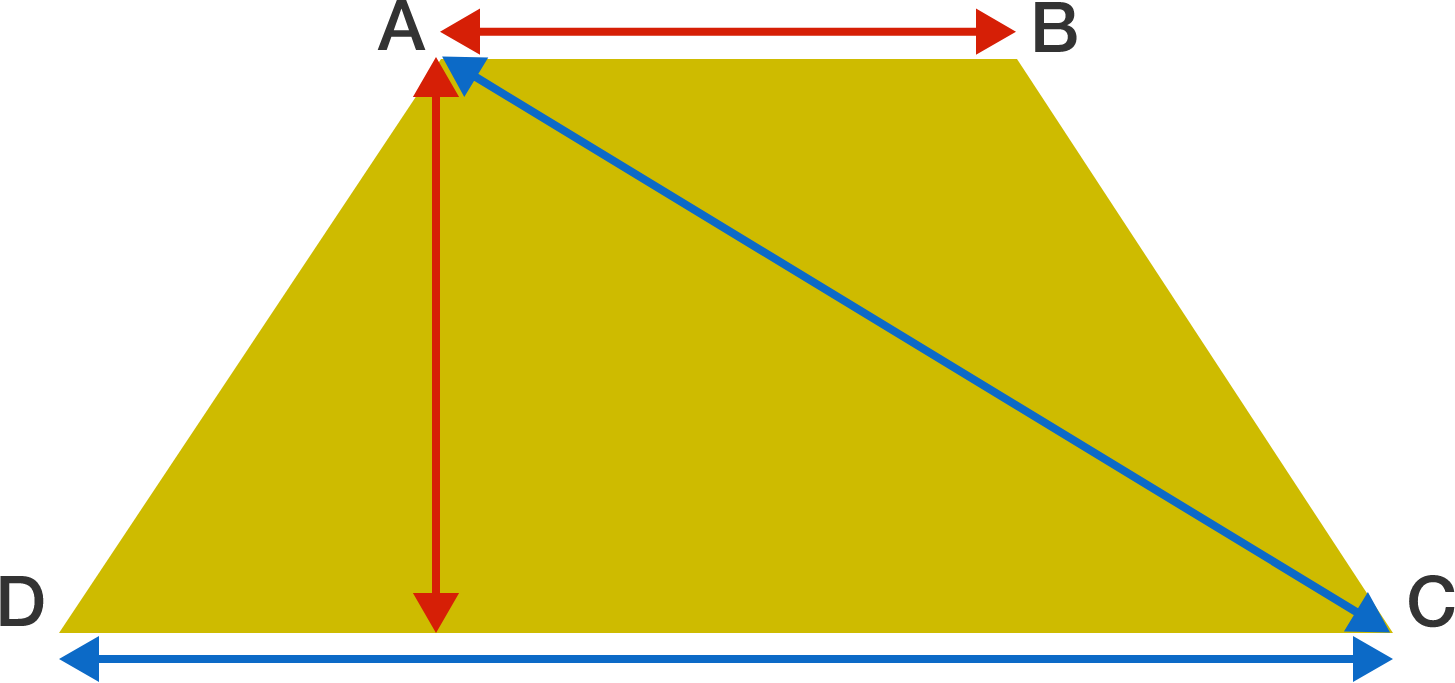Suppose $ABCD$ is an isosceles trapezoid with bases $AB$ and $CD$ and sides $AD$ and $BC$ such that $|CD| \gt |AB|.$ Also suppose that $|CD| = |AC|$ and that the altitude of the trapezoid is equal to $|AB|.$

If $\dfrac{|AB|}{|CD|} = \dfrac{a}{b},$ where $a$ and $b$ are positive coprime integers, then find $\large a^{b}.$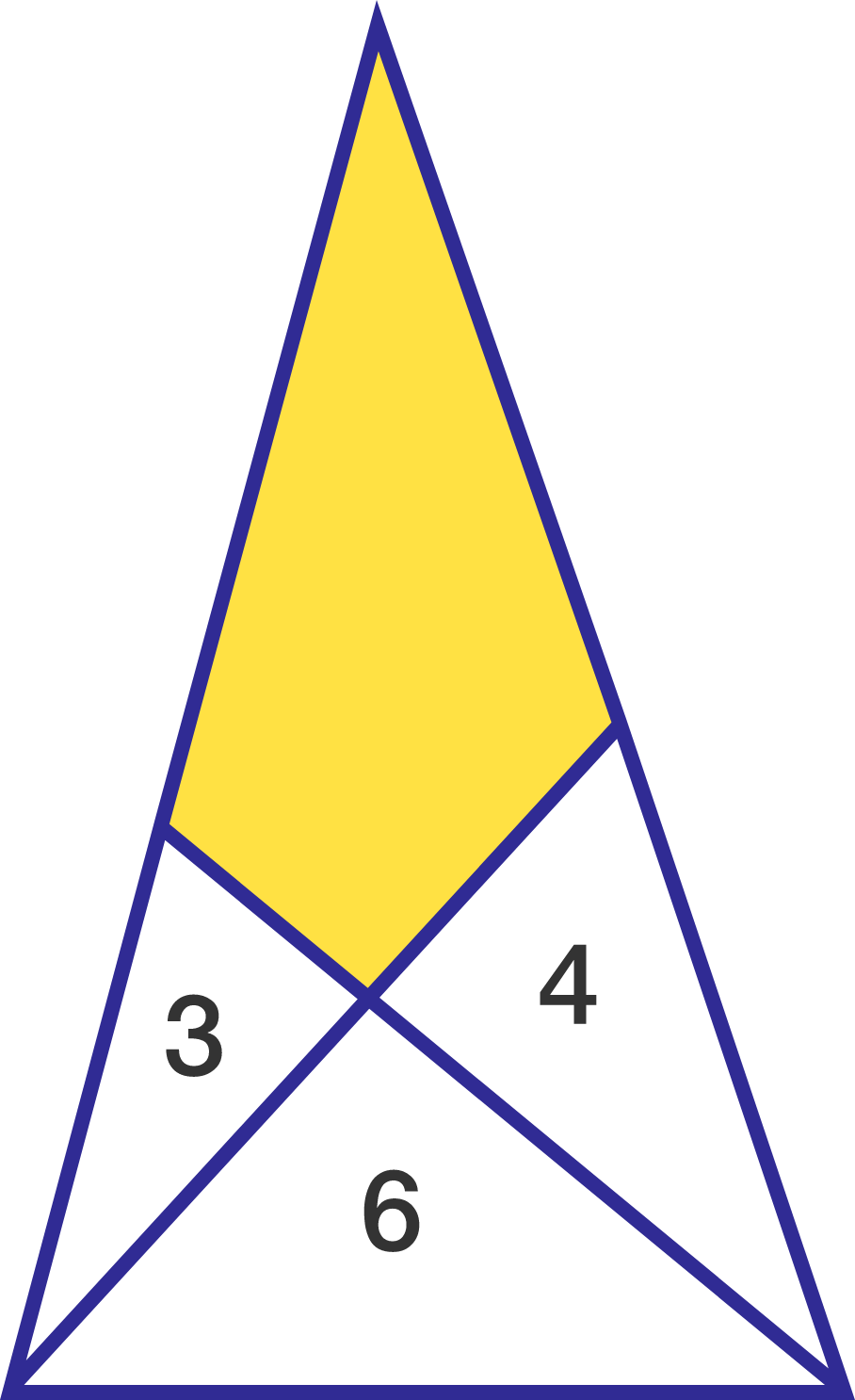The numbers $3,4,$ and $6$ denote the areas enclosed by their respective triangles.

What is the area of the yellow region?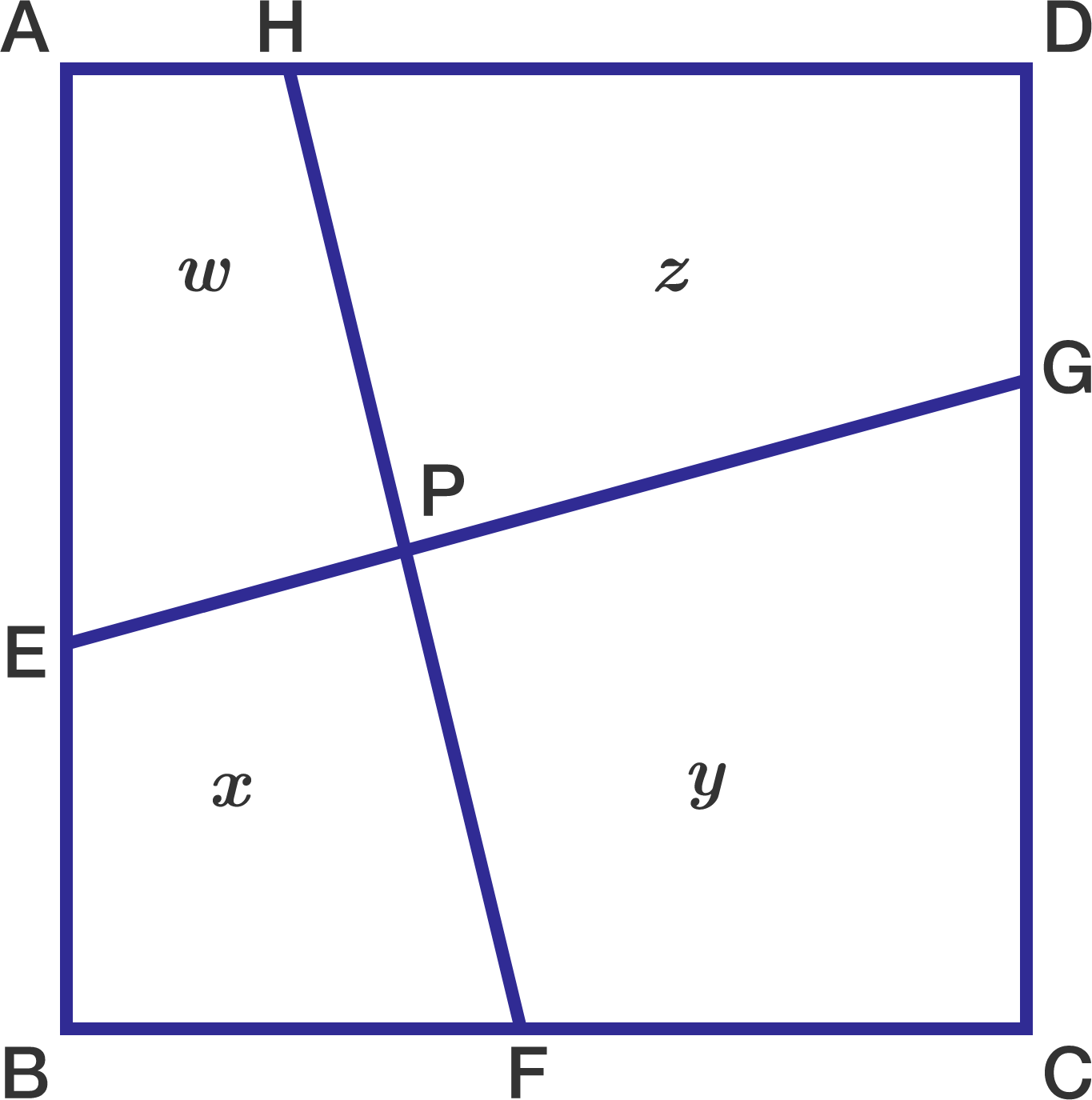On square $ABCD$, points $E,F,G$, and $H$ lie on sides $\overline{AB},\overline{BC},\overline{CD},$ and $\overline{DA},$ respectively, so that $\overline{EG} \perp \overline{FH}$ and $EG=FH = 34$. Segments $\overline{EG}$ and $\overline{FH}$ intersect at a point $P$, and the areas of the quadrilaterals $AEPH, BFPE, CGPF,$ and $DHPG$ are in the ratio $269:275:405:411.$ Find the area of square $ABCD$.I want to make a litter box for my newly adopted pet kitten Admiral. The box is a cuboid with the top removed. I want the volume of the box to be 32 but the surface area to be minimized.

What is the minimum internal surface area I can achieve?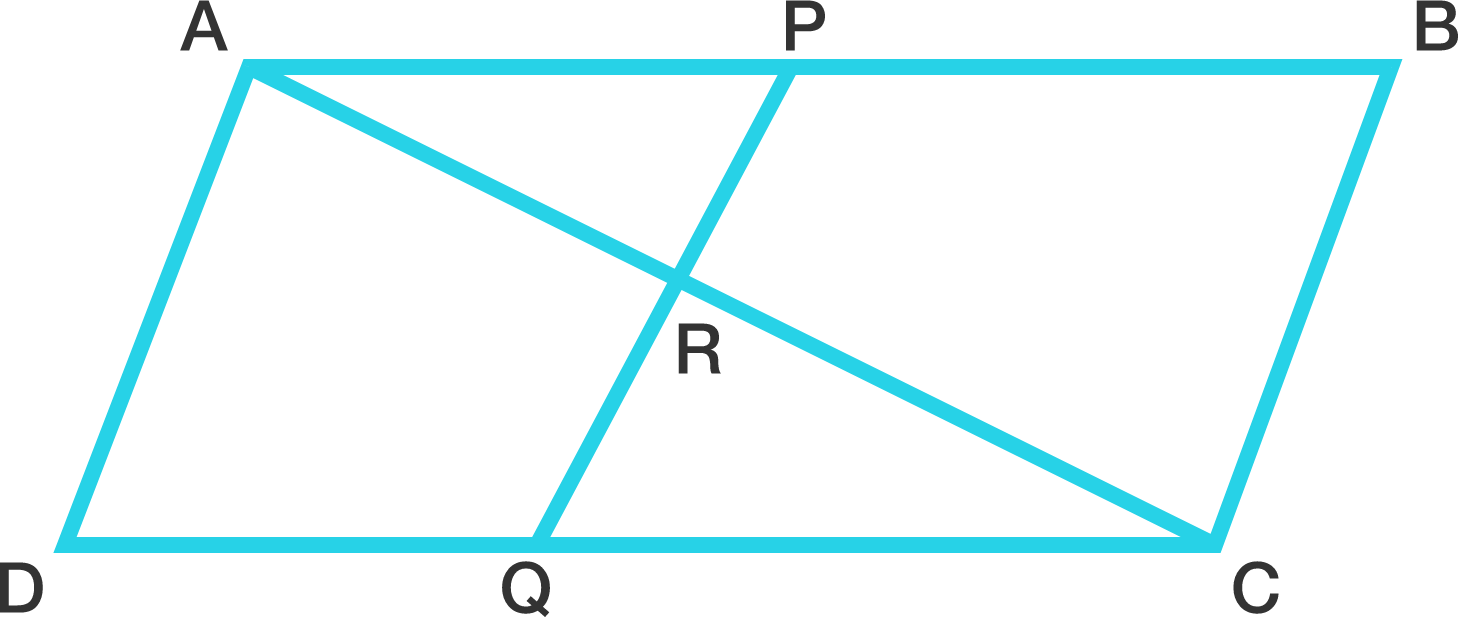$ABCD$ is a parallelogram. Point $P$ on $AB$ divides it in the ratio $AP : PB = 3 : 2$, and point $Q$ on $CD$ divides it in the ratio $CQ : QD = 7 : 3$. Let $R$ be the intersection of $PQ$ and $AC$. Then, $AR : AC = a : b$, where $a$ and $b$ are positive coprime integers. What is $a + b$?

×

Problem Loading...

Note Loading...

Set Loading...